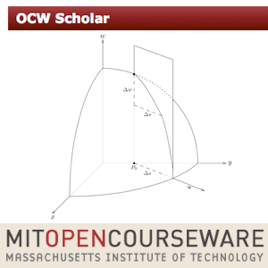70 episodios

This course covers differential, integral and vector calculus for functions of more than one variable. These mathematical tools and methods are used extensively in the physical sciences, engineering, economics and computer graphics.

OCW Scholar courses are designed for independent learners who have few additional resources available to them. The courses are substantially more complete than typical OCW courses and include new custom-created content as well as materials repurposed from MIT classrooms. The materials are are also arranged in logical sequences and include multimedia such as video and simulations.# OCW Scholar: Multivariable Calculus MIT

• Educación

This course covers differential, integral and vector calculus for functions of more than one variable. These mathematical tools and methods are used extensively in the physical sciences, engineering, economics and computer graphics.

OCW Scholar courses are designed for independent learners who have few additional resources available to them. The courses are substantially more complete than typical OCW courses and include new custom-created content as well as materials repurposed from MIT classrooms. The materials are are also arranged in logical sequences and include multimedia such as video and simulations.

• video
Coordinate free proofs: centroid of a triangle

## Coordinate free proofs: centroid of a triangle

This course covers differential, integral and vector calculus for functions of more than one variable. These mathematical tools and methods are used extensively in the physical sciences, engineering, economics and computer graphics.

• 11 min
• video
Dot products and angles

## Dot products and angles

This course covers differential, integral and vector calculus for functions of more than one variable. These mathematical tools and methods are used extensively in the physical sciences, engineering, economics and computer graphics.

• 8 min
• video
Components of a vector

## Components of a vector

This course covers differential, integral and vector calculus for functions of more than one variable. These mathematical tools and methods are used extensively in the physical sciences, engineering, economics and computer graphics.

• 5 min
• video
Area of a parallelogram

## Area of a parallelogram

This course covers differential, integral and vector calculus for functions of more than one variable. These mathematical tools and methods are used extensively in the physical sciences, engineering, economics and computer graphics.

• 3 min
• video
Determinants

## Determinants

This course covers differential, integral and vector calculus for functions of more than one variable. These mathematical tools and methods are used extensively in the physical sciences, engineering, economics and computer graphics.

• 4 min
• video
Volume of a parallelepiped

## Volume of a parallelepiped

This course covers differential, integral and vector calculus for functions of more than one variable. These mathematical tools and methods are used extensively in the physical sciences, engineering, economics and computer graphics.

• 2 min